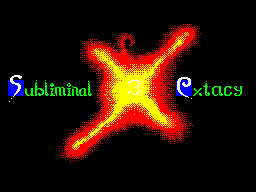Subliminal Extacy #03 01 апреля 2001# Draw Routine with Bresenham Algorithms```             Draw Routine with Bresenham Algorithms
Written by Mikezt

One of the most basic graphic routines is a line draw. When  you
try to write a draw routine  you may find it a bit  complicated.
Don't worry, here's one of way of doing it.

Bresenham algorithms have an advantage over methods because they
work with  integer numbers.  So they  can be  easily written  in
machine code.  Before we  start, get  ready for  some mathematic
expressions. I'll write lower indexes in [].

Abscissa is defined with two  points (x1, y1)  and  (x2, y2) and
can be written in the expression:

y = kx + q

where k is the direction and q is the shift on the y axis.

Direction  k   indicates  inclination   of  the line  and divide
coordination  system  to  eight parts  (see  pic. 1).  We  get a
situation  where k is positive  and less than 1.  Let's  presume
that point  (x[i], y[i])  was drawn.  For each  pixel we have to
compute  the  differences  from the  ideal  course  of  abscissa
(pic. 2).

Difference of both possible points are:
D1 = y - y[i] = k * (x[i] + 1) + q - y[i]
D2 = y[i] + 1 - y = y[i] + 1 - k * (x[i] + 1) - q
Difference of these two values is equal to:
D = D1 - D2 = 2 * k * (x[i] + 1) - 2 * y[i] + 2 * q - 1

Along this  difference we can choose the right point. It's clear
that  the factor of decision is it's sign. A negative value will
select the point (x[i] + 1, y[i] + 1),  and a positive  one will
select  (x[i] + 1, y[i]).  The disadvantage is real character of
values. We can remove this disadvantage  by  multiply difference
expression  by  (x2 - x1) and  we  get the  expression  which is
called decision:

d[i]=D*(x2-x1)=2*(y2-y1)*(x[i]+1)-2*y[i]*(x2-x1)+(2*q-1)*(x2-x1)

d[i]=2*(y2-y1)*x[i]-2*y[i]*(x2-x1)+2*(y2-y1)+(2*q-1)*(x2-x1)

Expression 2*(y2-y1)+(2*q-1)*(x2-x1) is constant and we indicate
it with c. so:

d[i] = 2 * (y2 - y1) * x[i] - 2 * y[i] * (x2 - x1) + c

Now this is an integer value, but it's quite complicated. If  we
express  decision for the  next point we can  write a  recursive
relation. Here is decision for the next point:

d[i+1] = 2*(y2 - y1)*(x[i] + 1) - 2*y[i+1]*(x2 - x1) + c

After  substracting  these  two  decisions we  can  express  the
decision value in a recursive form:

d[i+1] = d[i] + 2 * (y2 - y1) - 2 * (x2 - x1) * (y[i+1] - y[i])

Expression (y[i+1] - y[i]) can only store values 0 and 1 and  we
can write modified expressions to compute decision:

d[i] < 0   =>  d[i+1] = d[i] + 2 * (y2 - y1)
d[ ] >= 0  =>  d[i+1] = d[i] + 2 * (y2 - y1) - 2 * (x2 - x1)

Last thing you  need to know is the decision  for the first step
and here is:

d = 2 * (y2 - y1) - (x2 - x1)

So all  you must do  is check the  decision for  it's sign, if's
negative, move  the y coordinate, compute decision  for the next
step and test if you've drawn the  last point. If not, do it all
again. For  the other direction  that 0 x1  (or any other one  and choose  variants with
this comparison)  and if it's true  then there's no  change at
the start and end points.
- decision equations should be  divided by 4 (two  rotations) so
you can store it in an 8bit register, and no precision's lost.

Uf, that's all. My routine is available (I hope) on the HighTech
project (http://hightech.da.ru). I hope you understand what I've
tryed to say here. If not feel free to write to me. Bye!

mike/zt/ctl
jurica@sknet.sk```

Другие статьи номера: